Question

6. What would be the effect on measured cell potential if some solution in one well...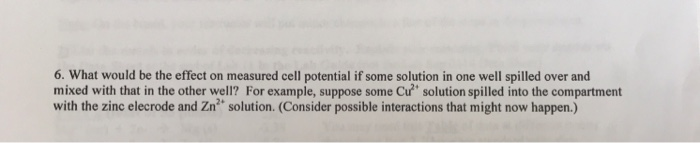6. What would be the effect on measured cell potential if some solution in one well spilled over and mixed with that in the other well? For example, suppose some Cu solution spilled into the compartment with the zinc elecrode and Zn solution. (Consider possible interactions that might now happen.)

Solution:

When some of the Cu2+ spilled into the compartment with Zn electrode and Zn2+ ions, then the metalic Cu is deposited on Zn electrode and hence, cell potential will be reduced.

Zn +Cu2+ = Zn2+ + Cu (metal)

This Cu metal deposited on Zn electrode and hence minimizes the cell potential.

Earn Coins

Coins can be redeemed for fabulous gifts.

Similar Homework Help Questions
• Do measured cell potentials show any effect of solution concentration on cell potential for the reactions...

Do measured cell potentials show any effect of solution concentration on cell potential for the reactions performed in this experiment? For example, would the potential have changed for 0.1 M solutions from standard conditions of 1 M solutions. Yes, the Nernst equation shows potential is dependent on solution concentrations. No, the Nernst equation does not show potential is dependent on solution concentrations. The potential would change if the cathode and anode were mislabeled. Potential is dependent on concentration, but in...

• A concentration cell is called such because both the anode and cathode are build from the same components, meaning the standard cell potential is zero volts and the measured cell potential entirely d...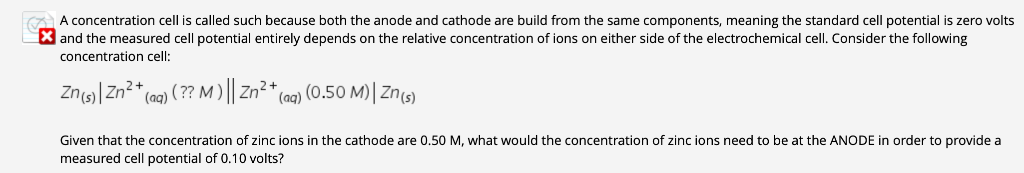A concentration cell is called such because both the anode and cathode are build from the same components, meaning the standard cell potential is zero volts and the measured cell potential entirely depends on the relative concentration of ions on either side of the electrochemical cell. Consider the following concentration cell Zns)lZn2() (77M)Zn2()(0.50 M)| Znø) 0.50 M) Zn Given that the concentration of zinc ions in the cathode are 0.50 M, what would the concentration of zinc ions need to...

• In a copper-zinc voltaic cell, one half-cell consists of a ZnZn electrode inserted in a solution...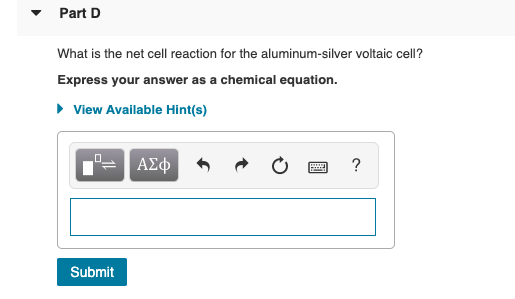In a copper-zinc voltaic cell, one half-cell consists of a ZnZn electrode inserted in a solution of zinc sulfate and the other half-cell consists of a CuCu electrode inserted in a copper sulfate solution. These two half-cells are separated by a salt bridge. At the zinc electrode (anode), ZnZn metal undergoes oxidation by losing two electrons and enters the solution as Zn2+Zn2+ ions. The oxidation half-cell reaction that takes place at the anode is Zn(s)→Zn2+(aq)+2e−Zn(s)→Zn2+(aq)+2e− The CuCu ions undergo reduction...

• Calculate the theoretical cell potential (E°) of a galvanic cell under standard conditions made up of...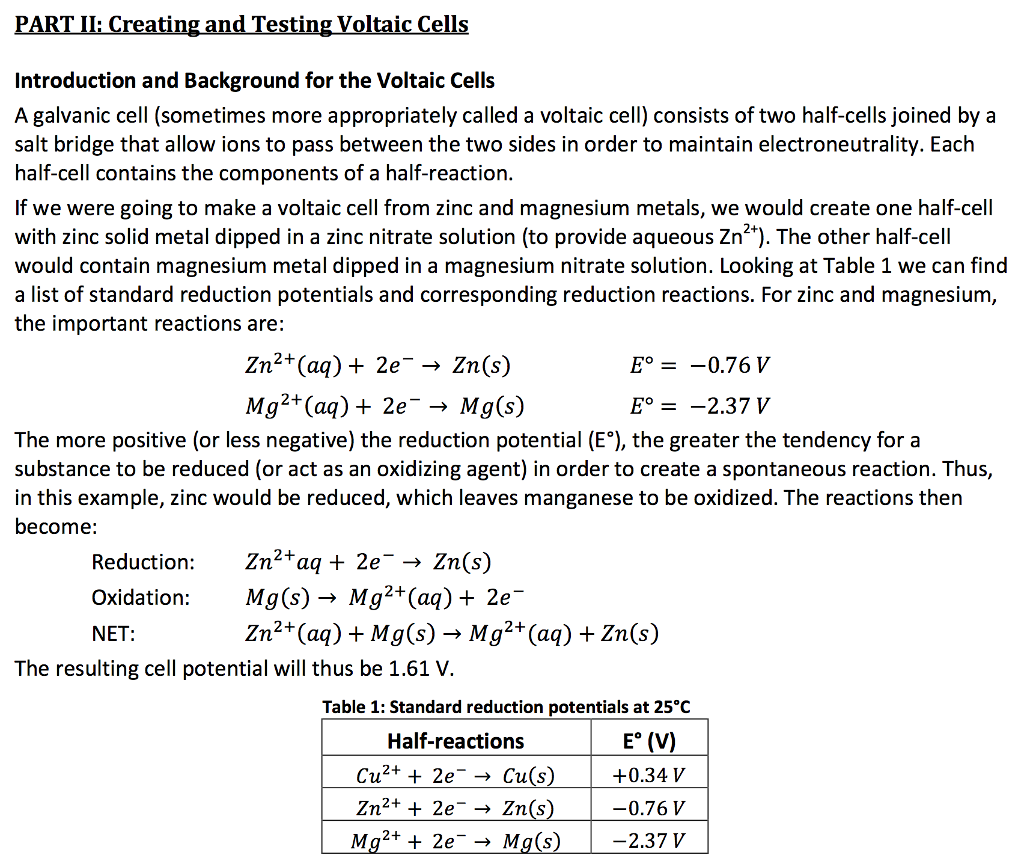Calculate the theoretical cell potential (E°) of a galvanic cell under standard conditions made up of copper and magnesium (see Part II and Table 1 for more information). PARTIL Creating and Testing Voltaic Cells Introduction and Background for the Voltaic Cells A galvanic cell (sometimes more appropriately called a voltaic cell) consists of two half-cells joined by a salt bridge that allow ions to pass between the two sides in order to maintain electroneutrality. Each half-cell contains the Components of...

• Prelab Questions: (PL1) For 6 galvanic cell combinations in part 1, write a chemical equation for each cell i...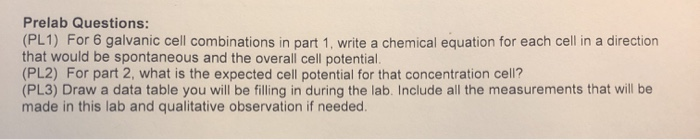Prelab Questions: (PL1) For 6 galvanic cell combinations in part 1, write a chemical equation for each cell in a direction that would be spontaneous and the overall cell potential (PL2) For part 2, what is the expected cell potential for that concentration cell? (PL3) Draw a data table you will be filling in during the lab. Include all the measurements that will be made in this lab and qualitative observation if needed. Procedure: Part 1) (1) Obtain copper strip,...

• EXPERIMENT 1: List the measured potential for Cell 3: Zn|Zn(NO3)2 || Pb(NO3)2|Pb. Based on your observations,...

EXPERIMENT 1: List the measured potential for Cell 3: Zn|Zn(NO3)2 || Pb(NO3)2|Pb. Based on your observations, do you expect given electrochemical cell to be spontaneous or nonspontaneous? Explain your answer. Pb|Pb(NO3)2||Zn|Zn(NO3)2   EXPERIMENT 1: What would happen to the measured cell potentials if 30 mL solution was used in each half-cell instead of 25 mL? EXPERIMENT 1: Calculate the theoretical standard cell potential for the electrochemical cell that includes the reaction. Mn+Pb2+⟶Mn2++Pb The standard reduction potentials for each half reaction are...

• What would happen to the measured cell potentials if 30 mL solution was used in each...

What would happen to the measured cell potentials if 30 mL solution was used in each half-cell instead of 25 mL?

• What would happen to the measured cell potentials if 30 mL solution was used in each...

What would happen to the measured cell potentials if 30 mL solution was used in each half-cell instead of 25 mL? will rate, thanks!

• 5. What was the purpose of the NaNO3 solution in this experiment? 6. Could a solution...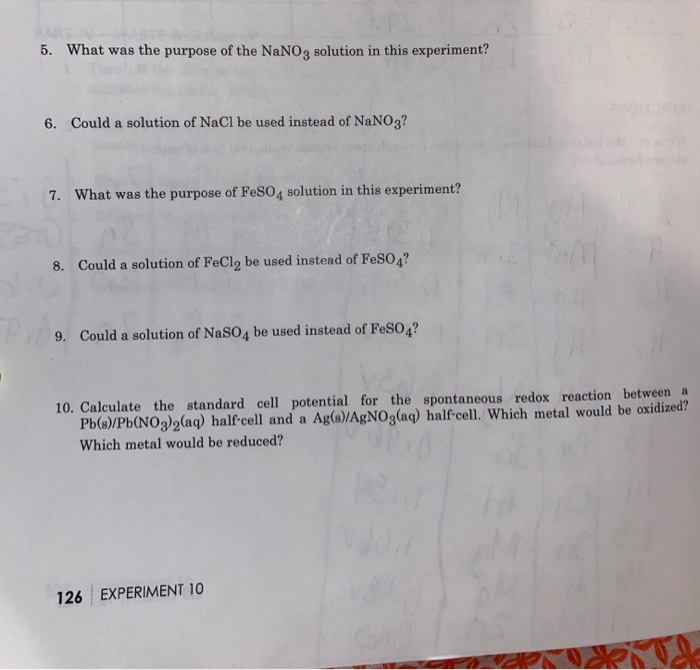5. What was the purpose of the NaNO3 solution in this experiment? 6. Could a solution of NaCl be used instead of NaNO3? 7. What was the purpose of FeSO4 solution in this experiment? 8. Could a solution of FeCl, be used instead of FeSO4? 9. Could a solution of NaSO4 be used instead of FeSO4? 10. Calculate the standard cell potential for the spontaneous redox reaction between a Pb(s)/Pb(NO3)2(aq) half-cell and a Ag(s)/AgNO3(aq) half-cell. Which metal would be oxidized?...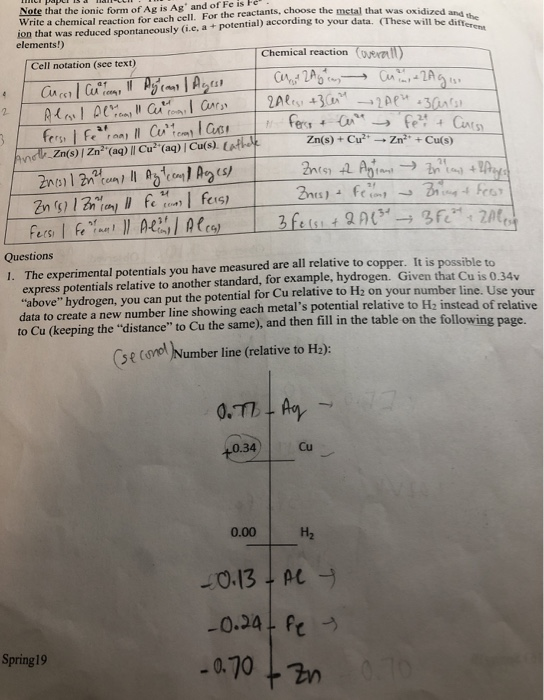Chemistry: Experimental Cell Potential Lab report please help checking my work and answering question #2 #3 #4 Thanks! *question #2 #3 #4* Note that the ionic form of Ag is Ag' and of Fe is Fe Write a chemical reaction for each cell. For the reactants, choose the metal that was oxidized ion that was reduced spontaneously (i.e, a + potential) according to your data. (Thesethe elements!) Chemical reaction (oall) Cell notation (see text) (NA1 Zn(s)+Cu-Zn+Cu(s) r凸の, Zn(s) | Zn2...